1800-1023-196

+91-120-4616500

# Type III

## Type III

In a molecule containing two or more atoms of same or different elements in different oxidation states.

(i) Oxidation number of S in Na2S2O3

By usual method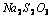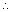2 × (+1) + 2 × x + 3 (–2) = 0 or 2 + 2x – 6 = 0

or x = 2.

But this is unacceptable as the two sulphur atoms in Na2S2O3 cannot have the same oxidation number because on treatment with dil. H2SO4, one sulphur atom is precipitated while the other is oxidised to SO2.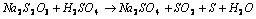In this case, the oxidation number of sulphur is evaluated from concepts of chemical bonding. The chemical structure of Na2S2O3 is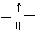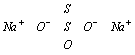Due to the presence of a co-ordinate bond between two sulphur atoms, the acceptor sulphur atom has oxidation number of – 2 whereas the other S atom gets oxidation number of + 2.

2 × (+1) + 3 × (–2) + x × 1 + 1 × (– 2) = 0

(for Na) (for O) (for S) (for coordinated S)

or + 2 – 6 + x – 2 = 0 or x = + 6

Thus two sulphur atoms in Na2S2O3 have oxidation number of – 2 and +6.

(ii) Oxidation number of chlorine in CaOCl2

(bleaching powder)

In bleaching powder, Ca(OCl)Cl, the two Cl atoms are in different oxidation states i.e., one Cl having oxidation number of –1 and the other as OCl having oxidation number of +1.

(iii) Oxidation number of N in NH4NO3

By usual method N2H4O3 ; 2x + 4 × (+1) + 3 × (–1) = 0

2x + 4 – 3 = 0 or 2x = + 1 (wrong)

No doubt NH4NO3 has two nitrogen atoms but one N has negative oxidation number (attached to H) and the other has positive oxidation number (attached to O). Hence the evaluation should be made separately for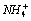and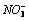x + 4 × (+1) = +1 or x = – 3x + 3 (– 2) = –1 or x = + 5.

(iv) Oxidation number of Fe in Fe3O4

In Fe3O4, Fe atoms are in two different oxidation states. Fe3O4 can be considered as an equimolar mixture of FeO [iron (II) oxide] and Fe2O3 [iron (III) oxide]. Thus in one molecule of Fe3O4, two Fe atoms are in + 3 oxidation state and one Fe atom is in + 2 oxidation state.

(v) Oxidation number of S in sodium tetrathionate (Na2S4O6)

Its structure can be represented as follows,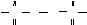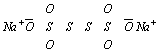The two S-atoms which are linked to each other have oxidation number zero. The oxidation number of other S-atoms can be calculated as follows

Let oxidation number of S = x.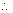2 × x + 2 × 0 + 6 × ( – 2) = – 2

(for S) (for S–S) (for O)

x = + 5.

## NEET & AIIMS Exam Sample Papers

 AIIMS SAMPLE PAPERS View More NEET SAMPLE PAPERS View More